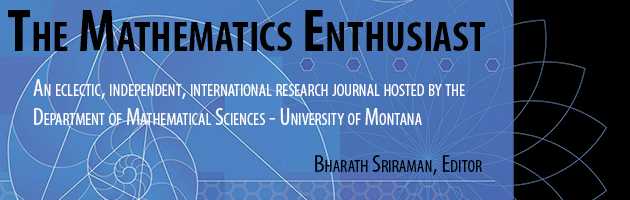•
•#### Article Title

Exploring Secondary Students' Modelling Competencies

17

1

#### Abstract

Mathematical modelling is a very important component in the teaching and learning of mathematics. In Malaysia, educators require more exposure to mathematical modelling it is still a new pedagogical method in classrooms. Modelling tasks that require students to construct a mathematical model would be a good start in developing modelling competencies. In this study, the authors investigate three mathematical modelling competencies of secondary students in the state of Selangor through a qualitative analysis of 20 students (divided into four groups) responses based on one modelling task developed by the researchers. The three modelling competencies we focus in this study are (1) making assumption; (2) computing and interpreting solution; and (3) mathematical reasoning. Our findings indicate that most students were basic users while a small percentage reached the proficient users level. With more engagement with the modelling tasks, students would able to acquire the modelling competencies and also develop their modelling skills. This would contribute to the meaningful learning of mathematics.

85

107

#### Digital Object Identifier (DOI)

10.54870/1551-3440.1481

COinS